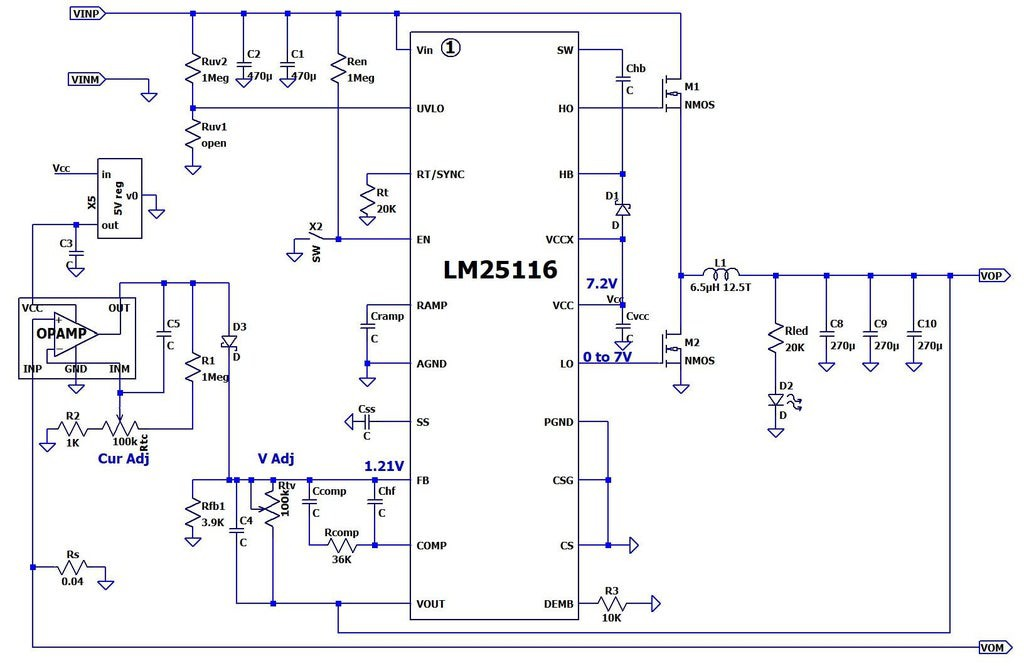# Ideas for digitizing the current control of SZBK07 DC-DC buck converter

I have the popular SZBK07 DC-DC buck converter, which seems to have the following schematics:The manufacturer gives the following parameters:

• Non-isolated, adjustable DC/DC buck (step down) constant-voltage or constant-current converter (supplies constant voltage until set current is reached, then constant current)
• Not reverse polarity protected
• Input/output voltage differential: 3 V min.
• Input voltage must be higher than required output voltage
• Input Voltage: DC 6-40 V (10 to 40 V is suggested)
• Output Voltage: DC 1.2-36 VDC
• Output Ripple: ~ 50 mV
• Rated Current: 15 A, 20 A max.
• NOTE: Wattage Limited 300 W max. (V x I x Eff. = W)
• Efficiency: 96% max (Nominal 86%
• Current Adj. (CC): sets the available charging/driving current
• Operating Temperature: -10°C to 75°C (Fan recommened if heatsink > 65°C)
• Working frequency: 150 kHz

My goal is to use this converter as a regulated constant-current source, which I need to control digitally. I will use it at a fixed input voltage of about 12 V. The output current range that I need is from the lowest possible up to about 7 A (it could be little bit higher if I can get a little bit higher voltage from the power source).

As can be seen from the schematics, the current control is executed in the following way: the voltage drop over the shunt Rs = 0.004 Ω (wrong value in the picture) is amplified by an op-amp (LM321) and the output voltage is fed through D3 to the FB input of the LM25116, where it is combined with the voltage from the voltage control circuit (Rfb1 - Rtv). So both control the same thing - the output voltage.

So my ideas to digitize the current control are:

1. Exchange Rtc with a digital 100kΩ potentiometer. The problem is that I cannot find such potentiometer available nearby, but I can find 10 kΩ. So the questions here are: Is there some general problem using a digital potentiometer? Can I decrease the values of R2, Rtc and R1 (for example 10 times) in order to use a 10kΩ potentiometer or to recalculate them to fit 10 kΩ in the range of control that I want?

2. In case there is some problem with the previous idea, I could remove D3, measure the output of the opamp with the microcontroller (10-bits ADC) and control the voltage Vadj with PWM or DAC. Any problems with this? Probably problems when the DAC or PWM outputs are not initialized yet?

3. Instead of using the op-amp output, I can measure the current with a Hall sensor and use it in the same way as in point 2.

Ideas and advice are highly appreciated.

• The schematic is missing a connection from M1 source to SW, and probably CS/CSG to a shunt resistor below M2. Operating in voltage mode is strongly discouraged. The simplest way to add external control is wire a resistor from DAC output to FB, leaving Rtv fixed. This keeps compensation constant, at least as long as supply voltage is in nominal range (loop gain is proportional to supply). Note D3 introduces a tempco to the current setpoint. Apr 13 at 11:43
• Yes, you are right about the missing M1 source (M2 drain) to SW connection. It exists on the board. However, CS, CSG and M2 source are connected directly to ground and there is no shunt resistor. The only shunt is Rs (lower left corner) - you can see that the output "VON" is not ground, but it is "lifted" from it by Rs. So the opamp measure the drop over it. So, if I understand correctly, your proposal is to leave everything as is and just add a DAC output to FB? But how to measure the current? And what does it mean "Operating in voltage mode is strongly discouraged"? Apr 13 at 12:58
• Datasheet: ti.com/cn/lit/ds/symlink/lm25116.pdf?ts=1681367131223 Read the sections on ramp generator, current limit, etc., and familiarize yourself with peak current mode operation, and voltage mode operation. The basics are: this device is designed to operate with a per-cycle current sense, and V(COMP) corresponds to the inductor current; the error amp controls inductor current to regulate output voltage (or current as the case may be). Going directly from VFB to PWM ("voltage mode") incurs two poles, making an unstable (or at least difficult to stabilize) system. Apr 13 at 13:32
• I assume disabling current sense on this device will have an effect akin to voltage mode in traditional controllers e.g. TL494, but maybe it won't work at all, I don't know. Apr 13 at 13:33
• @Tim Williams I changed the Rtc potentiometer with a digital one, (10k + 100k in series, to optimize the lower range) and it works, but not exactly as I expect. I power two peltiers in series and when I set a potentiometer to a given value I expect it to keep the same current no matter the changes of the Peltiers resistance, but in reality the current is not constant.. For example at the same potentiometer value the current starts at 5.2A, and after 10 minutes it falls to 4.7A. I don't know why it behaves like this. Jun 23 at 10:47